Open in App
Not now

# Flutter – Stepper Widget

• Last Updated : 18 Nov, 2021

In this article, we will learn about the Stepper widget in Flutter. A stepper widget displays progress through a sequence of steps. Stepper is generally used in filling forms online.

For example, remember filling an online form for applying to any university or passport or driving license. We filled the form step by step like

• In step 1 we have filled in our personal details
• In step 2 we have entered our residence address
• In step 3 we have given  our education details
• In step 4 we did payment
• In step 5 we got registered and print the receipt

This is actually called a Stepper. Performing the task in a step-by-step process.

Now let’s see the practical implementation of our stepper widget:

Step 1: Open a new flutter project in your editor.

Step 2: In scaffold, we initialized Stepper() and inside stepper, we created a method called stepList(). This method is created because we have created the list of steps that are required in the form.

## Dart

 `import ``'package:flutter/material.dart'``;`   `void` `main() {` `  ``runApp(``const` `MyApp());` `}`   `class` `MyApp extends StatelessWidget {` `  ``const` `MyApp({Key? key}) : super(key: key);`   `  ``// This widget is the root of your application.` `  ``@override` `  ``Widget build(BuildContext context) {` `    ``return` `MaterialApp(` `      ``title: ``'Flutter Demo'``,` `      ``theme: ThemeData(` `        ``primarySwatch: Colors.blue,` `      ``),` `      ``home: ``const` `MyHomePage(),` `    ``);` `  ``}` `}`   `class` `MyHomePage extends StatefulWidget {` `  ``const` `MyHomePage({Key? key}) : super(key: key);`   `  ``@override` `  ``_MyHomePageState createState() => _MyHomePageState();` `}`   `class` `_MyHomePageState extends State {` `  `  `  ``// Here we have created list of steps that` `  ``// are required to complete the form` `   ``List stepList() => [` `        ``const` `Step(title: Text(``'Account'``), content: Center(child: Text(``'Account'``),)),` `         ``const` `Step(title: Text(``'Address'``), content: Center(child: Text(``'Address'``),)),` `          ``const` `Step(title: Text(``'Confirm'``), content: Center(child: Text(``'Confirm'``),))` `   ``];` `  ``@override` `  ``Widget build(BuildContext context) {` `    ``return` `Scaffold(` `      ``appBar: AppBar(` `        ``centerTitle: ``true``,` `        ``backgroundColor: Colors.green,` `        ``title: ``const` `Text(``'GeeksforGeeks'``,style: TextStyle(color: Colors.white), ),` `      ``),` `      `  `      ``// Here we have initialized the stepper widget ` `      ``body: Stepper(` `        ``steps: stepList(),` `      ``)` `    ``);` `  ``}` `}`

Output: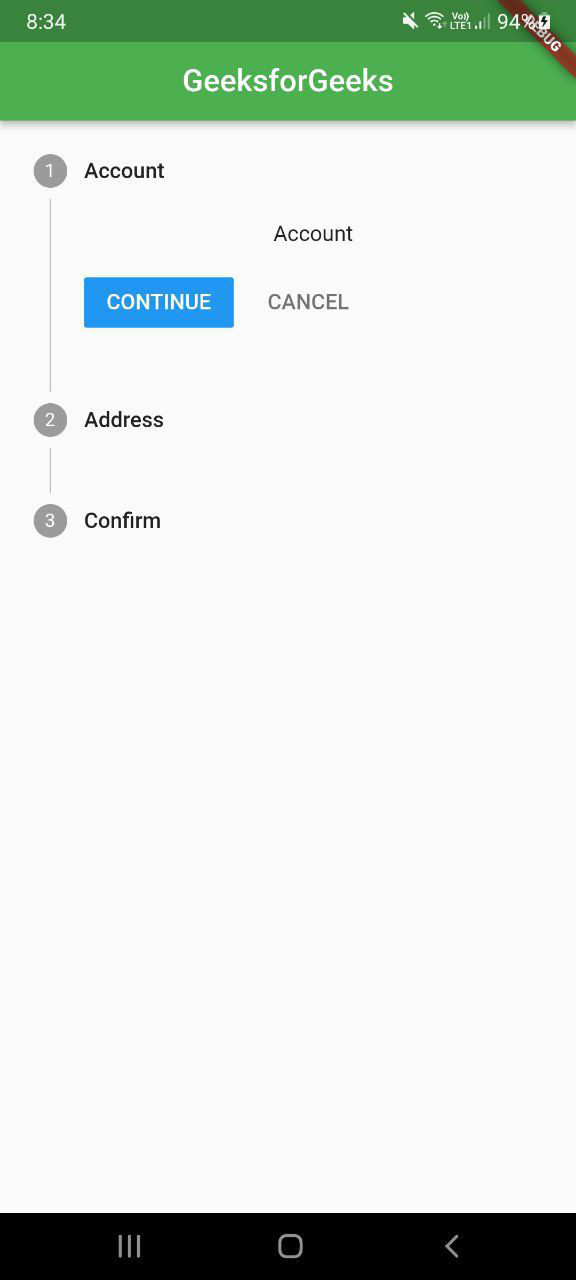Note: Now, this is the basic Stepper widget. If we want we can change the stepper in horizontal form as well. Just type the command in the below picture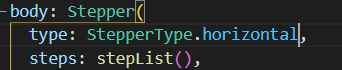Output: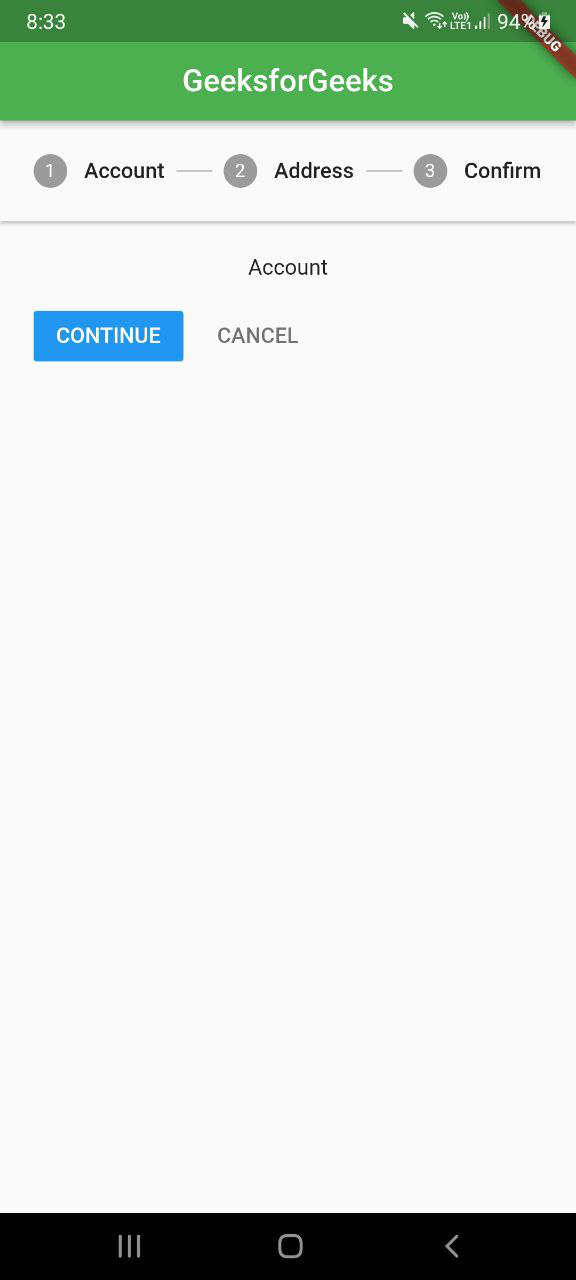Step 3: Now let’s add properties to this Stepper Widget like –

• currentStep: We have created an integer. Its initial value is 0. It actually shows the content of the step on which that particular index is.
• onStepContinue:  It’s the callback called when the continue button is tapped. When we click on the continue button it takes us to the next step. If null, the ‘continue’ button will be disabled
• onStepCancel: It’s the callback called when the cancel button is tapped. When we click on the cancel button then we push back to the previous step. If null, the ‘cancel’ button will be disabled.
• onStepTapped: Callback called when the step is tapped. We can go directly to any step by just clicking on that particular step. for eg – if you want to go directly on step2 then just click on it and you will be there.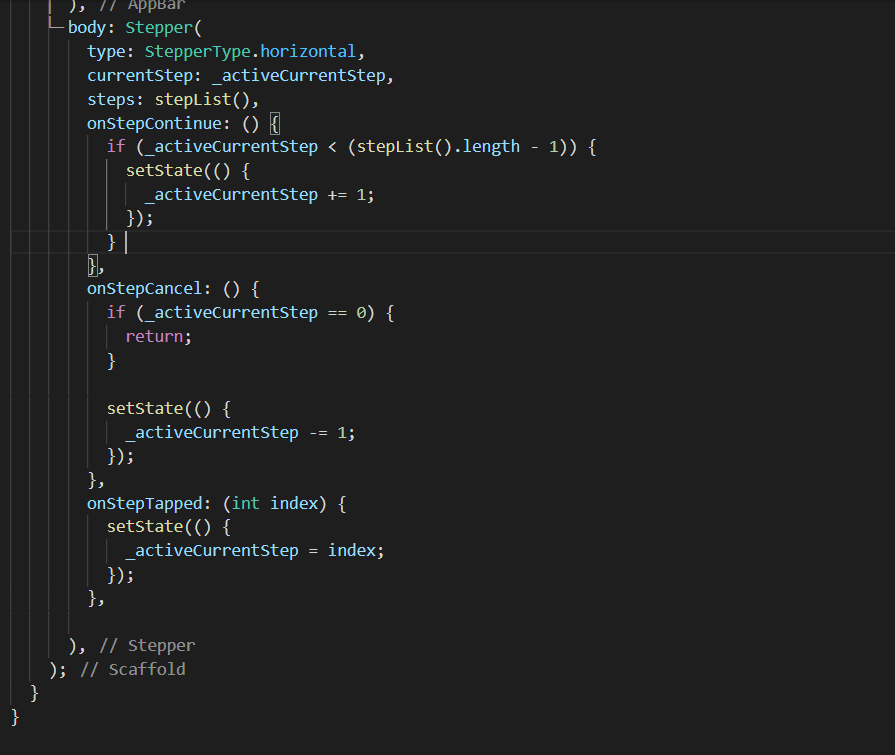Step 4: In this step, we had created a form by adding textfields so that the user can input data. So it basically looks more like a form-like structure.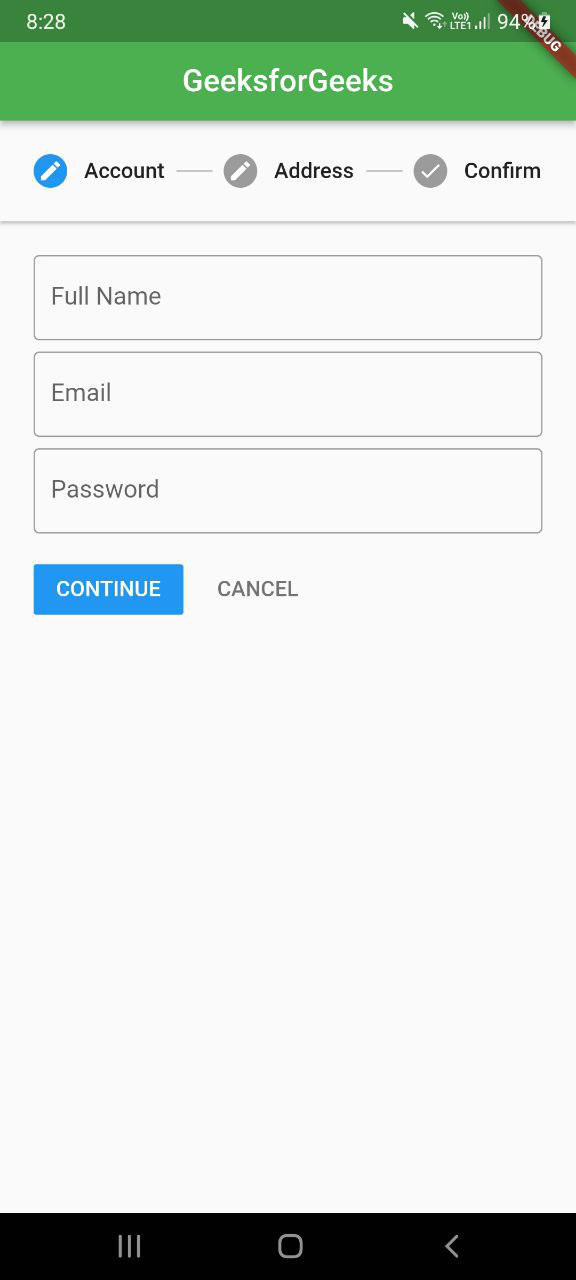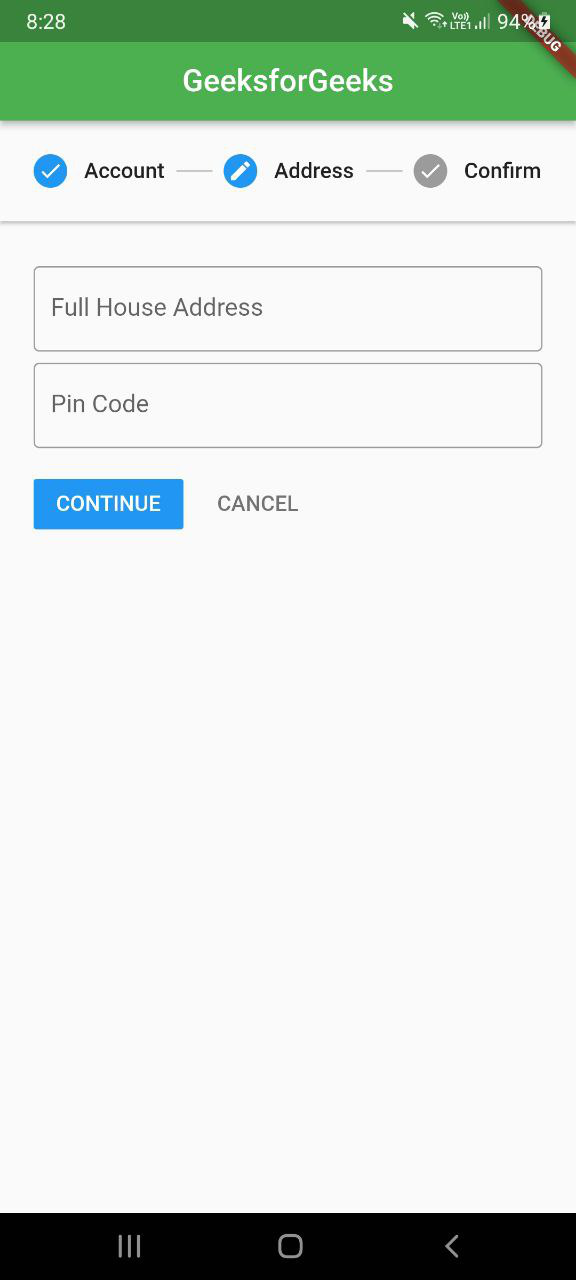• In the third step Confirm we are displaying data that the user has entered.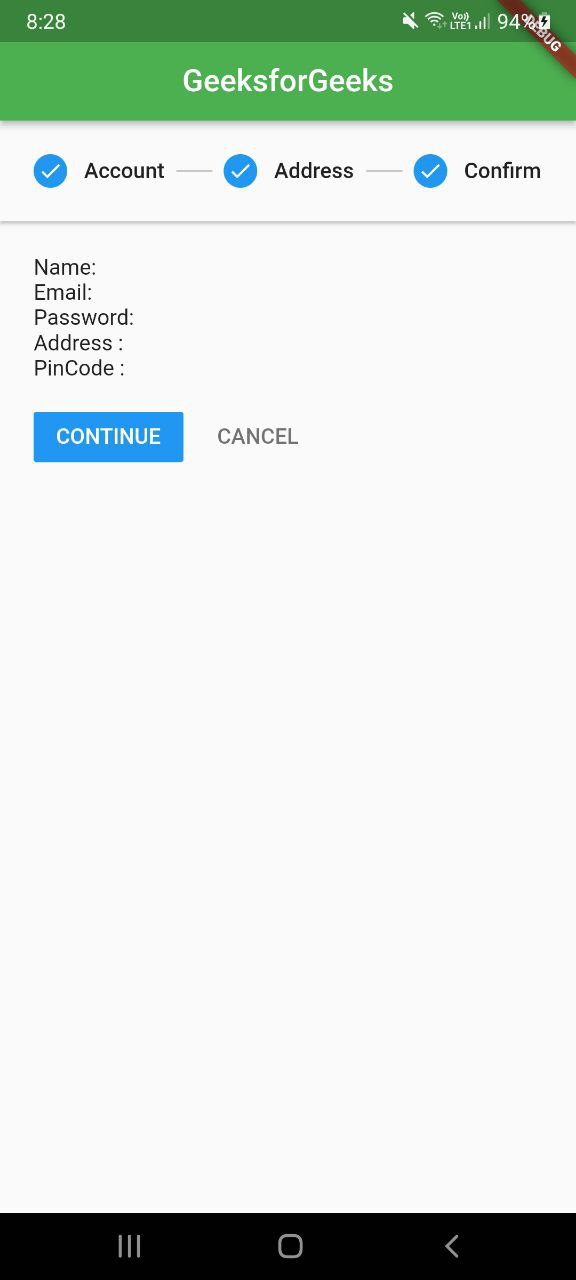Full Source Code:

## Dart

 `import ``'package:flutter/material.dart'``;`   `void` `main() {` `  ``runApp(``const` `MyApp());` `}import ``'package:flutter/material.dart'``;`   `void` `main() {` `  ``runApp(``const` `MyApp());` `}`   `class` `MyApp extends StatelessWidget {` `  ``const` `MyApp({Key? key}) : super(key: key);`   `  ``// This widget is the root of your application.` `  ``@override` `  ``Widget build(BuildContext context) {` `    ``return` `MaterialApp(` `      ``title: ``'Flutter Demo'``,` `      ``theme: ThemeData(` `        ``primarySwatch: Colors.blue,` `      ``),` `      ``home: ``const` `MyHomePage(),` `    ``);` `  ``}` `}`   `class` `MyHomePage extends StatefulWidget {` `  ``const` `MyHomePage({Key? key}) : super(key: key);`   `  ``@override` `  ``_MyHomePageState createState() => _MyHomePageState();` `}`   `class` `_MyHomePageState extends State {` `  `  `  ``// we have initialized active step to 0 so that` `  ``// our stepper widget will start from first step ` `  ``int` `_activeCurrentStep = 0;`   `  ``TextEditingController name = TextEditingController();` `  ``TextEditingController email = TextEditingController();` `  ``TextEditingController pass = TextEditingController();` `  ``TextEditingController address = TextEditingController();` `  ``TextEditingController pincode = TextEditingController();` `  `  `  ``// Here we have created list of steps` `  ``// that are required to complete the form` `  ``List stepList() => [` `     ``// This is step1 which is called Account.` `     ``// Here we will fill our personal details` `        ``Step(` `          ``state: _activeCurrentStep <= 0 ? StepState.editing : StepState.complete,` `          ``isActive: _activeCurrentStep >= 0,` `          ``title: ``const` `Text(``'Account'``),` `          ``content: Container(` `            ``child: Column(` `              ``children: [` `                ``TextField(` `                  ``controller: name,` `                  ``decoration: ``const` `InputDecoration(` `                    ``border: OutlineInputBorder(),` `                    ``labelText: ``'Full Name'``,` `                  ``),` `                ``),` `                ``const` `SizedBox(` `                  ``height: 8,` `                ``),` `                ``TextField(` `                  ``controller: email,` `                  ``decoration: ``const` `InputDecoration(` `                    ``border: OutlineInputBorder(),` `                    ``labelText: ``'Email'``,` `                  ``),` `                ``),` `                ``const` `SizedBox(` `                  ``height: 8,` `                ``),` `                ``TextField(` `                  ``controller: pass,` `                  ``obscureText: ``true``,` `                  ``decoration: ``const` `InputDecoration(` `                    ``border: OutlineInputBorder(),` `                    ``labelText: ``'Password'``,` `                  ``),` `                ``),` `              ``],` `            ``),` `          ``),` `        ``),` `        ``// This is Step2 here we will enter our address` `        ``Step(` `            ``state:` `                ``_activeCurrentStep <= 1 ? StepState.editing : StepState.complete,` `            ``isActive: _activeCurrentStep >= 1,` `            ``title: ``const` `Text(``'Address'``),` `            ``content: Container(` `              ``child: Column(` `                ``children: [` `                  ``const` `SizedBox(` `                    ``height: 8,` `                  ``),` `                  ``TextField(` `                    ``controller: address,` `                    ``decoration: ``const` `InputDecoration(` `                      ``border: OutlineInputBorder(),` `                      ``labelText: ``'Full House Address'``,` `                    ``),` `                  ``),` `                  ``const` `SizedBox(` `                    ``height: 8,` `                  ``),` `                  ``TextField(` `                    ``controller: pincode,` `                    ``decoration: ``const` `InputDecoration(` `                      ``border: OutlineInputBorder(),` `                      ``labelText: ``'Pin Code'``,` `                    ``),` `                  ``),` `                ``],` `              ``),` `            ``)),` `    `  `            ``// This is Step3 here we will display all the details` `            ``// that are entered by the user` `        ``Step(` `            ``state: StepState.complete,` `            ``isActive: _activeCurrentStep >= 2,` `            ``title: ``const` `Text(``'Confirm'``),` `            ``content: Container(` `                ``child: Column(` `              ``crossAxisAlignment: CrossAxisAlignment.stretch,` `              ``mainAxisAlignment: MainAxisAlignment.start,` `              ``children: [` `                ``Text(``'Name: \${name.text}'``),` `                ``Text(``'Email: \${email.text}'``),` `                ``Text(``'Password: \${pass.text}'``),` `                ``Text(``'Address : \${address.text}'``),` `                ``Text(``'PinCode : \${pincode.text}'``),` `              ``],` `            ``)))` `      ``];` `  `  `  ``@override` `  ``Widget build(BuildContext context) {` `    ``return` `Scaffold(` `      ``appBar: AppBar(` `        ``centerTitle: ``true``,` `        ``backgroundColor: Colors.green,` `        ``title: ``const` `Text(``'GeeksforGeeks'``,style: TextStyle(color: Colors.white), ),` `      ``),` `      ``// Here we have initialized the stepper widget` `      ``body: Stepper(` `        ``type: StepperType.horizontal, ` `        ``currentStep: _activeCurrentStep,` `        ``steps: stepList(),` `        `  `        ``// onStepContinue takes us to the next step` `        ``onStepContinue: () {` `          ``if` `(_activeCurrentStep < (stepList().length - 1)) {` `            ``setState(() {` `              ``_activeCurrentStep += 1;` `            ``});` `          ``} ` `        ``},` `        `  `        ``// onStepCancel takes us to the previous step` `        ``onStepCancel: () {` `          ``if` `(_activeCurrentStep == 0) {` `            ``return``;` `          ``}`   `          ``setState(() {` `            ``_activeCurrentStep -= 1;` `          ``});` `        ``},` `        `  `        ``// onStepTap allows to directly click on the particular step we want` `        ``onStepTapped: (``int` `index) {` `          ``setState(() {` `            ``_activeCurrentStep = index;` `          ``});` `        ``},` `        `  `      ``),` `    ``);` `  ``}` `}`     `class` `MyApp extends StatelessWidget {` `  ``const` `MyApp({Key? key}) : super(key: key);`   `  ``// This widget is the root of your application.` `  ``@override` `  ``Widget build(BuildContext context) {` `    ``return` `MaterialApp(` `      ``title: ``'Flutter Demo'``,` `      ``theme: ThemeData(` `        ``primarySwatch: Colors.blue,` `      ``),` `      ``home: ``const` `MyHomePage(),` `    ``);` `  ``}` `}`   `class` `MyHomePage extends StatefulWidget {` `  ``const` `MyHomePage({Key? key}) : super(key: key);`   `  ``@override` `  ``_MyHomePageState createState() => _MyHomePageState();` `}`   `class` `_MyHomePageState extends State {` `  ``int` `_activeCurrentStep = 0;`   `  ``TextEditingController name = TextEditingController();` `  ``TextEditingController email = TextEditingController();` `  ``TextEditingController pass = TextEditingController();` `  ``TextEditingController address = TextEditingController();` `  ``TextEditingController pincode = TextEditingController();`   `  ``List stepList() => [` `        ``Step(` `          ``state: _activeCurrentStep <= 0 ? StepState.editing : StepState.complete,` `          ``isActive: _activeCurrentStep >= 0,` `          ``title: ``const` `Text(``'Account'``),` `          ``content: Container(` `            ``child: Column(` `              ``children: [` `                ``TextField(` `                  ``controller: name,` `                  ``decoration: ``const` `InputDecoration(` `                    ``border: OutlineInputBorder(),` `                    ``labelText: ``'Full Name'``,` `                  ``),` `                ``),` `                ``const` `SizedBox(` `                  ``height: 8,` `                ``),` `                ``TextField(` `                  ``controller: email,` `                  ``decoration: ``const` `InputDecoration(` `                    ``border: OutlineInputBorder(),` `                    ``labelText: ``'Email'``,` `                  ``),` `                ``),` `                ``const` `SizedBox(` `                  ``height: 8,` `                ``),` `                ``TextField(` `                  ``controller: pass,` `                  ``obscureText: ``true``,` `                  ``decoration: ``const` `InputDecoration(` `                    ``border: OutlineInputBorder(),` `                    ``labelText: ``'Password'``,` `                  ``),` `                ``),` `              ``],` `            ``),` `          ``),` `        ``),` `        ``Step(` `            ``state:` `                ``_activeCurrentStep <= 1 ? StepState.editing : StepState.complete,` `            ``isActive: _activeCurrentStep >= 1,` `            ``title: ``const` `Text(``'Address'``),` `            ``content: Container(` `              ``child: Column(` `                ``children: [` `                  ``const` `SizedBox(` `                    ``height: 8,` `                  ``),` `                  ``TextField(` `                    ``controller: address,` `                    ``decoration: ``const` `InputDecoration(` `                      ``border: OutlineInputBorder(),` `                      ``labelText: ``'Full House Address'``,` `                    ``),` `                  ``),` `                  ``const` `SizedBox(` `                    ``height: 8,` `                  ``),` `                  ``TextField(` `                    ``controller: pincode,` `                    ``decoration: ``const` `InputDecoration(` `                      ``border: OutlineInputBorder(),` `                      ``labelText: ``'Pin Code'``,` `                    ``),` `                  ``),` `                ``],` `              ``),` `            ``)),` `        ``Step(` `            ``state: StepState.complete,` `            ``isActive: _activeCurrentStep >= 2,` `            ``title: ``const` `Text(``'Confirm'``),` `            ``content: Container(` `                ``child: Column(` `              ``crossAxisAlignment: CrossAxisAlignment.stretch,` `              ``mainAxisAlignment: MainAxisAlignment.start,` `              ``children: [` `                ``Text(``'Name: \${name.text}'``),` `                ``Text(``'Email: \${email.text}'``),` `                ``Text(``'Password: \${pass.text}'``),` `                ``Text(``'Address : \${address.text}'``),` `                ``Text(``'PinCode : \${pincode.text}'``),` `              ``],` `            ``)))` `      ``];` `  `  `  ``@override` `  ``Widget build(BuildContext context) {` `    ``return` `Scaffold(` `      ``appBar: AppBar(` `        ``title: ``const` `Text(``'Flutter Stepper'``),` `      ``),` `      ``body: Stepper(` `        ``type: StepperType.horizontal,` `        ``currentStep: _activeCurrentStep,` `        ``steps: stepList(),` `        ``onStepContinue: () {` `          ``if` `(_activeCurrentStep < (stepList().length - 1)) {` `            ``setState(() {` `              ``_activeCurrentStep += 1;` `            ``});` `          ``} ` `        ``},` `        ``onStepCancel: () {` `          ``if` `(_activeCurrentStep == 0) {` `            ``return``;` `          ``}`   `          ``setState(() {` `            ``_activeCurrentStep -= 1;` `          ``});` `        ``},` `        ``onStepTapped: (``int` `index) {` `          ``setState(() {` `            ``_activeCurrentStep = index;` `          ``});` `        ``},` `        `  `      ``),` `    ``);` `  ``}` `}`

Output:

Explanation – In this video, we can see that:

• When we click on the continue button then we are heading towards the next step which means our onStepContinue property is working.
• When we click on the cancel button we are getting back to the previous step which means our onStepCancel property is working.
• When we tap on a particular step then we get straight directly to that particular step which means our onStepTapped property is working.

My Personal Notes arrow_drop_up
Related Articles